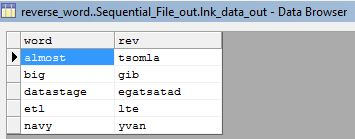# DATASTAGE SCENARIO: REVERSE THE WORDIn this DataStage scenario, we need to get the output as reverse letters in a given word.

almost
big
datastage
etl
navy

tsomla
gib
tle
yvan

## Solution:

Read the data using sequential file stage.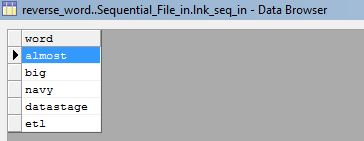We can achieve the required output by using 2 transformer stages like below.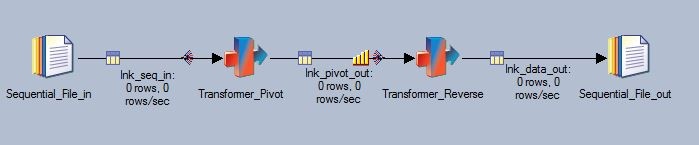In first transformer stage, define the stage variables like shown below.

Len() is a function to calculate the length of a field. So scount holds the value ‘6’ for ‘almost’ word and ‘3’ for ‘big’ word and so on.
In loop condition, define

@ITERATION <=scount

define loop variable ‘rev’ as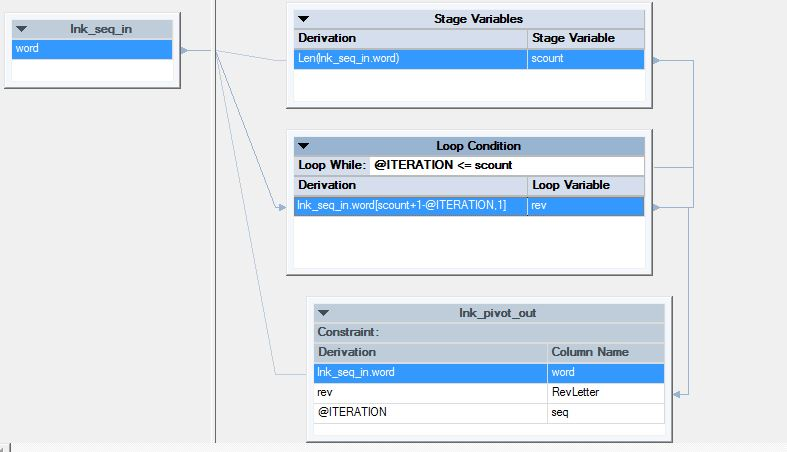If you are a DataStage user, you’ll probably know that there is a new “Loop Condition” section (since DataStage 8.5 version) on the transformer canvas.

You will notice there is a loop test (which is a “While” condition) that controls how many times the output logic should be iterated over.

The logic that has been shown includes a new variable named “@ITERATION” which is a counter indicating what pass through the loop this is.

One other item that will appear new are the Loop variables – basically the same as stage variables, but these get evaluated each time through the loop.

The rest of this logic is old like basic substringing and calculations that we do in derivations area.

After this first transformer stage, your data would look like:In second transformer stage, define the stage variables like shown below.

NumSavedRows=SavedInputRecord()
IsBreak=LastRowInGroup(lnk_pivot_out.word)
FinalWord=IF IsBreak THEN AddingWord:lnk_pivot_out.RevLetter ELSE “”
NumRows=IF IsBreak THEN NumSavedRows ELSE 0

SaveInputRecord():

This function saves the entire record in cache and returns the number of records that are currently stored in cache, starting from 1.

LastRowInGroup(InputColumn):

You can call this function when processing an input row, and the function returns TRUE if this row is the last row in the input data.

And Loop condition as

@ITERATION <=NumRows

In Constraints

@ITERATION=1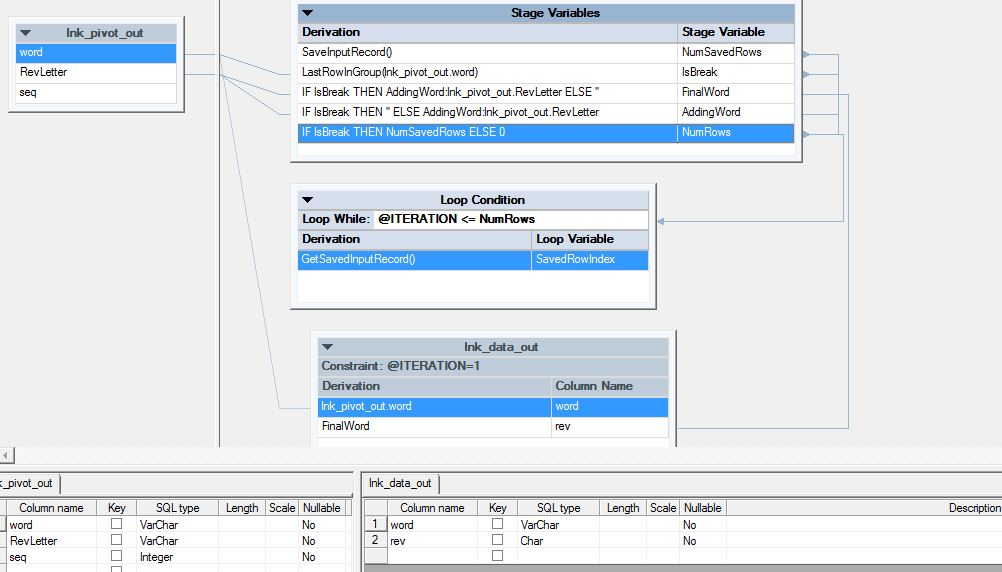At the same time, run this transformer stage in Sequential mode by having Inline sort on ‘word’ and ‘seq’ as keys. And keep ‘seq’ sort in ascending order.

Run the job.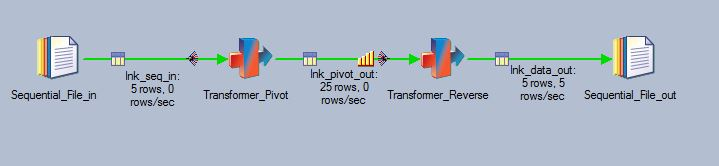Your final output would be like: